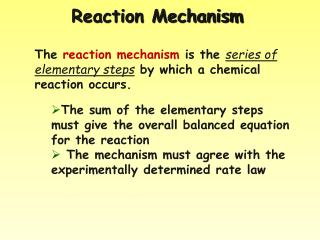DownloadDownload PresentationReaction Mechanism

# Reaction Mechanism

Télécharger la présentation## Reaction Mechanism

- - - - - - - - - - - - - - - - - - - - - - - - - - - E N D - - - - - - - - - - - - - - - - - - - - - - - - - - -
##### Presentation Transcript

1. Reaction Mechanism The reaction mechanism is the series of elementary steps by which a chemical reaction occurs. • The sum of the elementary steps must give the overall balanced equation for the reaction • The mechanism must agree with the experimentally determined rate law

2. A classic nucleophilic substitution mechanism

3. Rate-Determining Step In a multi-step reaction, the slowest step is the rate-determining step. It therefore determines the rate of the reaction. The experimental rate law must agree with the rate-determining step

4. Identifying the Rate-Determining Step For the reaction: 2H2(g) + 2NO(g)  N2(g) + 2H2O(g) The experimental rate law is: R = k[NO]2[H2] Which step in the reaction mechanism is the rate-determining (slowest) step? Step #1H2(g) + 2NO(g)  N2O(g) + H2O(g) Step #2N2O(g) + H2(g)  N2(g) + H2O(g) Step #1agrees with the experimental rate law

5. Identifying Intermediates For the reaction: 2H2(g) + 2NO(g)  N2(g) + 2H2O(g) Which species in the reaction mechanism are intermediates (do not show up in the final, balanced equation?) Step #1 H2(g) + 2NO(g)  N2O(g) + H2O(g) Step #2 N2O(g) + H2(g)  N2(g) + H2O(g) 2H2(g) + 2NO(g)  N2(g) + 2H2O(g)  N2O(g) is an intermediate

6. Collision Model Key Idea: Molecules must collide to react. However, only a small fraction of collisions produces a reaction. Why?

7. Collision Model Collisions must have sufficient energyto produce the reaction (must equal or exceed the activation energy). Colliding particles must be correctly orientedto one another in order to produce a reaction.

8. Factors Affecting Rate Increasing temperature always increases the rate of a reaction. • Particles collide more frequently • Particles collide more energetically Increasing surface area increases the rate of a reaction Increasing Concentration USUALLY increases the rate of a reaction Presence of Catalysts, which lower the activation energy by providing alternate pathways